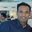Question-and-Answer Resource for the Building Energy Modeling Community
Get started with the Help page

# Default Condenser Loop Tower and Evaporative Fluid Cooler Design water flow rate

// Design water flow rate is assumed to be 3 gpm per ton (SI equivalent 5.382E-8 m3/s per watt)


For this to be true, this assumes that a ton is a Ton of Refrigeration (TR), or 12,000 BTU/h.

But in general cooling towers capacities are given in terms of Ton of Heat Rejection (THR), which at 3 GPM/ton gives 15,000 BTU/h.

Indeed, at Standard rating condition, the deltaT is 10°R, which gives:

$$1 THR= 3 {GPM} * 8.33 BTU/gal * 60 s/min * 10 R =15,000 BTU/h = 4396.07 W$$

$$3 GPM = 0.000189271 m^3/s$$

$$0.000189271 / 4396.07 = 4.305E-08 m^3.s^-1.W^-1$$

Why is 5.382E-8 m3/s per watt used insead of 4.305E-8 m3/s per watt?

So this means I have to calculate the High Speed Standard Design Capacity, it means I should use the manufacturer's THR and multiply it by 12,000 BTU/h?

What am I missing?

edit retag close merge delete

Sort by » oldest newest most votedTHR calculated above is 1.25*TR which is as expected due to compressor heat. (CTI document - Page 17/62)

From the source code, Tower nominal capacity in EnergyPlus doesn't include compressor heat. Standard Design or Nominal capacity is TR only. As the documentation also says that

The "Heat Rejection Capacity and Nominal Capacity Sizing Ratio" set in the previous field is applied to this capacity to give the actual fluid cooler heat rejection at these operating conditions.

Tower nominal capacity used to be multiplied by a constant factor of 1.25 to calculate UA. Now this factor can be specified by the user using the input field mentioned above.

Tower inputs in EnergyPlus should be as shown in this catalog data. CTI uses GPM/TR and not GPM/THR and that is why EnergyPlus uses 5.382E-8 m3/s per watt used insead of 4.305E-8 m3/s per watt.

more

And that's where I disagree. The catalog data gives tons in THR. 29-424 nominal tons, 87 to 1272 GPM. Do the calc, you'll see that nominal tons is 15,000 BTU/h.

This is also consistent with: "Product capacities are called out in terms of nominal tons. A nominal cooling tower ton is defined as the capability to cool 3 USGPM (0.19 l/s) of water from a 95ºF (35.0ºC) entering water temperature to an 85ºF (29.4ºC) leaving water temperature at a 78ºF (25.6ºC) entering wet-bulb temperature."

Refer to note in the idd for Nominal Capacity input filed in CoolingTower:SingleSpeed. It is TR and not THR. Catalog data shows 29-424 nominal tons for 87 to 1272 GPMs from simple 3 GPM/Ton rule. While 87GPM∗8.33BTU/gal∗60min/h∗10F/12000 give 36.24 (i.e. 29*1.25) and same is true for all other GPMs. The confusion here is because Nominal Capacity is thought as 36.24 Ton while EnergyPlus expects it as 29 Ton and 1.25 should specified in the input field in Heat Rejection Capacity and Nominal Capacity Sizing Ratio. Design tower load within E+ will then be calculated as 1.25 * 29 = 36.24.